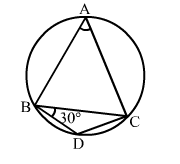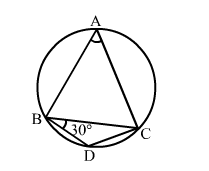# In the given figure, BD = DC and ∠CBD = 30°, find m(∠BAC).

Question:

In the given figure, BD = DC and CBD = 30°, find m(∠BAC).Solution:BD = DC
⇒ BCD = CBD = 30°
In ΔBCD, we have:
BCD + CBD + CDB = 180°  (Angle sum property of a triangle)
⇒ 30° + 30° + CDB = 180°
⇒ CDB = (180° – 60°) = 120°
The opposite angles of a cyclic quadrilateral are supplementary.
Thus, CDB + BAC = 180°
⇒ 120° + BAC = 180°
⇒ BAC = (180° – 120°) = 60°
∴ BAC = 60°